## 5-3 Zero Crossing Rate (嚙踝蕭嚙踝蕭嚙踝蕭嚙踝蕭嚙?

[chinese][all]

Zero-crossing rate (ZCR) is another basic acoustic feature that can be computed easily. It is equal to the number of zero-crossing of the waveform within a given frame. ZCR has the following characteristics:

• ZCR of unvoiced sounds and environmental noise are usually larger than voiced sounds, which has observable fundamental periods.
• It is hard to distinguish unvoiced sounds from environmental noise by using ZCR alone since they have similar ZCR values.
• ZCR is often used in conjunction with energy (or volume) for end-point detection. In particular, ZCR is used for detecting the start and end positions of unvoiced sounds.
• Some people use ZCR for rough fundamental frequency estimation, but it is highly unreliable unless further refine procedure is taken for post-processing.
To avoid DC bias, usually we need to perform mean subtraction on each frame. Here is an straightforward example of ZCR:

Example 1: zcr01.mwaveFile='csNthu.wav'; frameSize=256; overlap=0; au=myAudioRead(waveFile); y=au.signal; fs=au.fs; frameMat=enframe(y, frameSize, overlap); frameNum=size(frameMat, 2); for i=1:frameNum frameMat(:,i)=frameMat(:,i)-mean(frameMat(:,i)); % mean justification end zcr=sum(frameMat(1:end-1, :).*frameMat(2:end, :)<0); sampleTime=(1:length(y))/fs; frameTime=((0:frameNum-1)*(frameSize-overlap)+0.5*frameSize)/fs; subplot(2,1,1); plot(sampleTime, y); ylabel('Amplitude'); title(waveFile); subplot(2,1,2); plot(frameTime, zcr, '.-'); xlabel('Time (sec)'); ylabel('Count'); title('ZCR');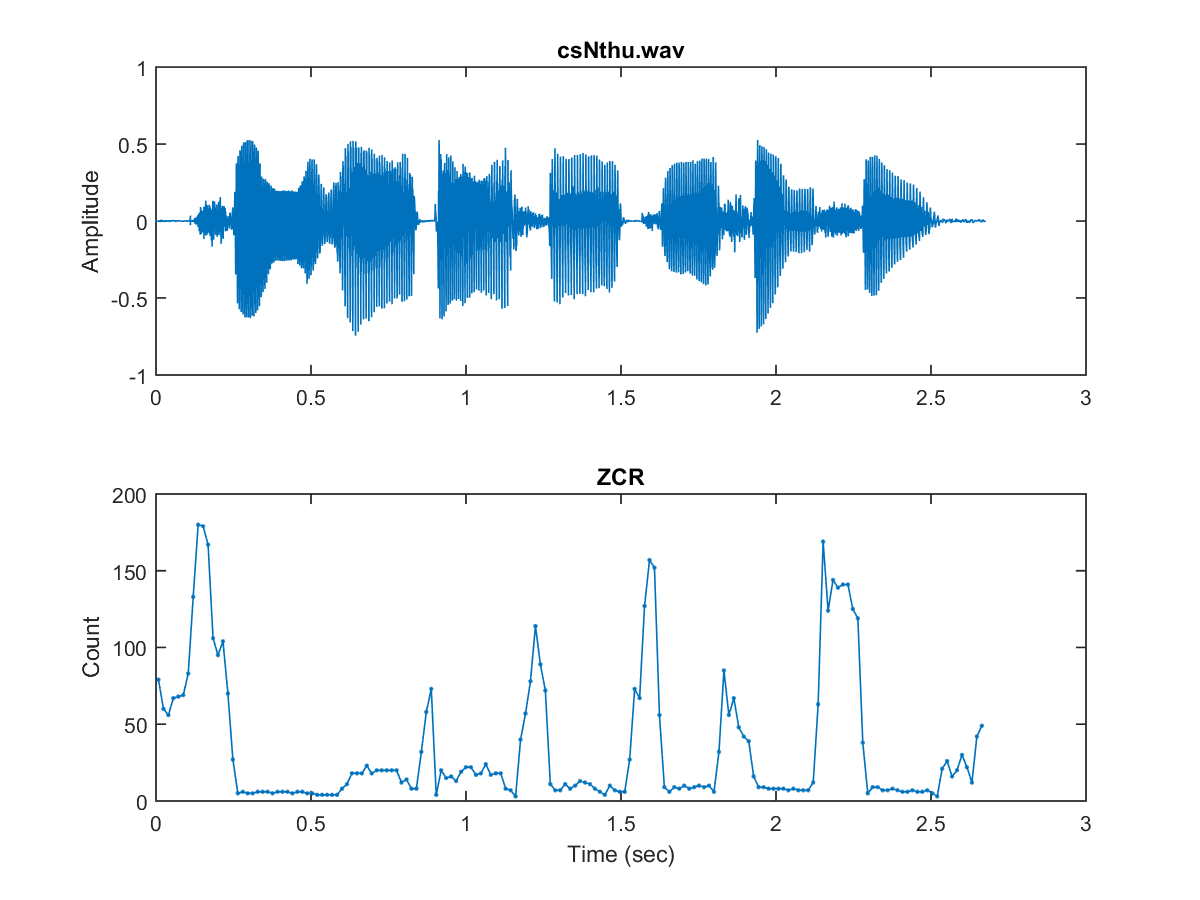We can use the function "frame2zcr" to simplify the above example:

Example 2: zcr02.mwaveFile='csNthu.wav'; frameSize=256; overlap=0; au=myAudioRead(waveFile); y=au.signal; fs=au.fs; frameMat=enframe(y, frameSize, overlap); frameNum=size(frameMat, 2); zcr=frame2zcr(frameMat); sampleTime=(1:length(y))/fs; frameTime=frame2sampleIndex(1:frameNum, frameSize, overlap)/fs; subplot(2,1,1); plot(sampleTime, y); ylabel('Amplitude'); title(waveFile); subplot(2,1,2); plot(frameTime, zcr, '.-'); xlabel('Time (sec)'); ylabel('Count'); title('ZCR');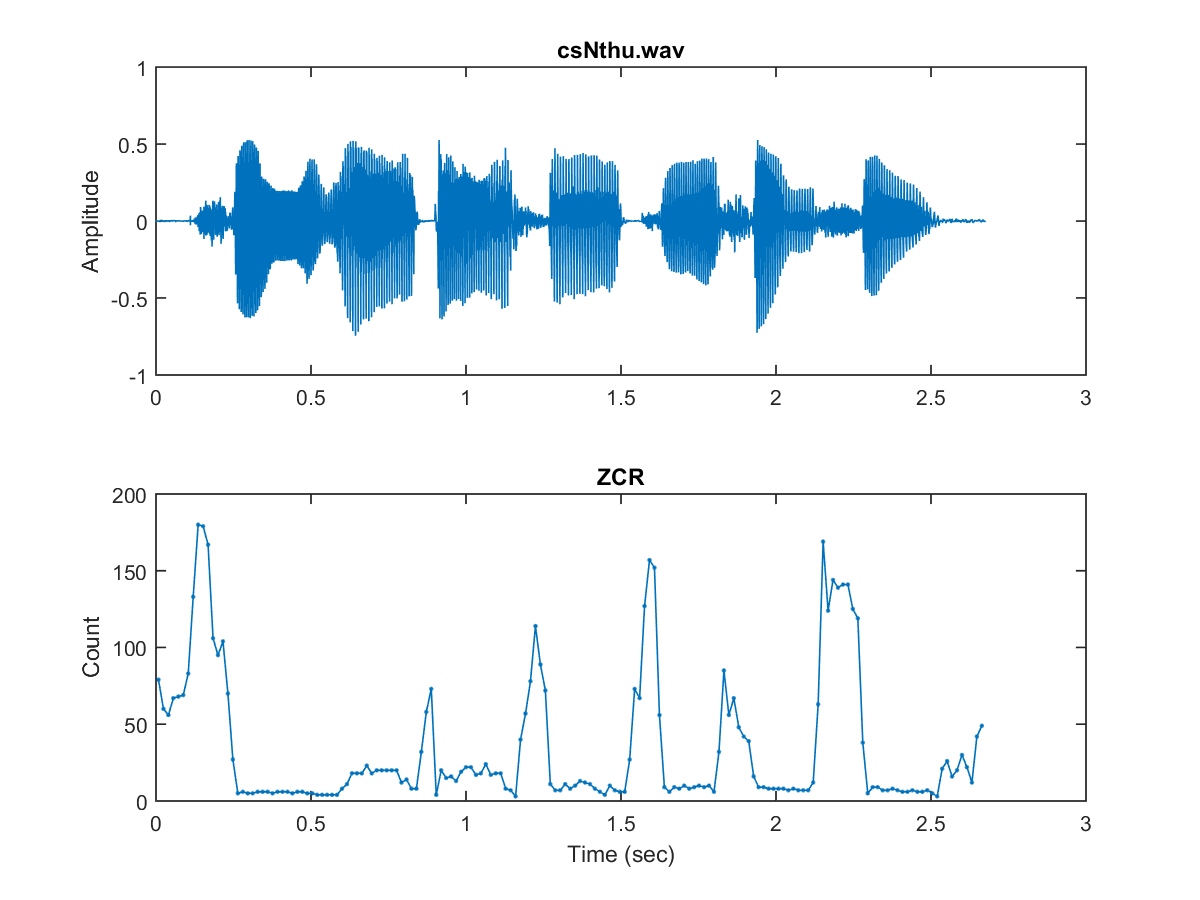In the above example, methods 1 and 2 return similar ZCR curves. In order to use ZCR to distinguish unvoiced sounds from environmental noise, we can shift the waveform before computing ZCR. This is particular useful is the noise is not too big. Example follows:

Example 3: zcrWithShift.mwaveFile='csNthu.wav'; frameSize=256; overlap=0; au=myAudioRead(waveFile); y=au.signal; fs=au.fs; frameMat=enframe(y, frameSize, overlap); frameNum=size(frameMat,2); volume=frame2volume(frameMat); [minVolume, index]=min(volume); shiftAmount=2*max(abs(frameMat(:,index))); % shiftAmount is equal to twice the max. abs. sample value within the frame of min. volume method=1; zcr1=frame2zcr(frameMat, method); zcr2=frame2zcr(frameMat, method, shiftAmount); sampleTime=(1:length(y))/fs; frameTime=frame2sampleIndex(1:frameNum, frameSize, overlap)/fs; subplot(2,1,1); plot(sampleTime, y); ylabel('Amplitude'); title(waveFile); subplot(2,1,2); plot(frameTime, zcr1, '.-', frameTime, zcr2, '.-'); xlabel('Time (sec)'); ylabel('Count'); title('ZCR'); legend('ZCR without shift', 'ZCR with shift');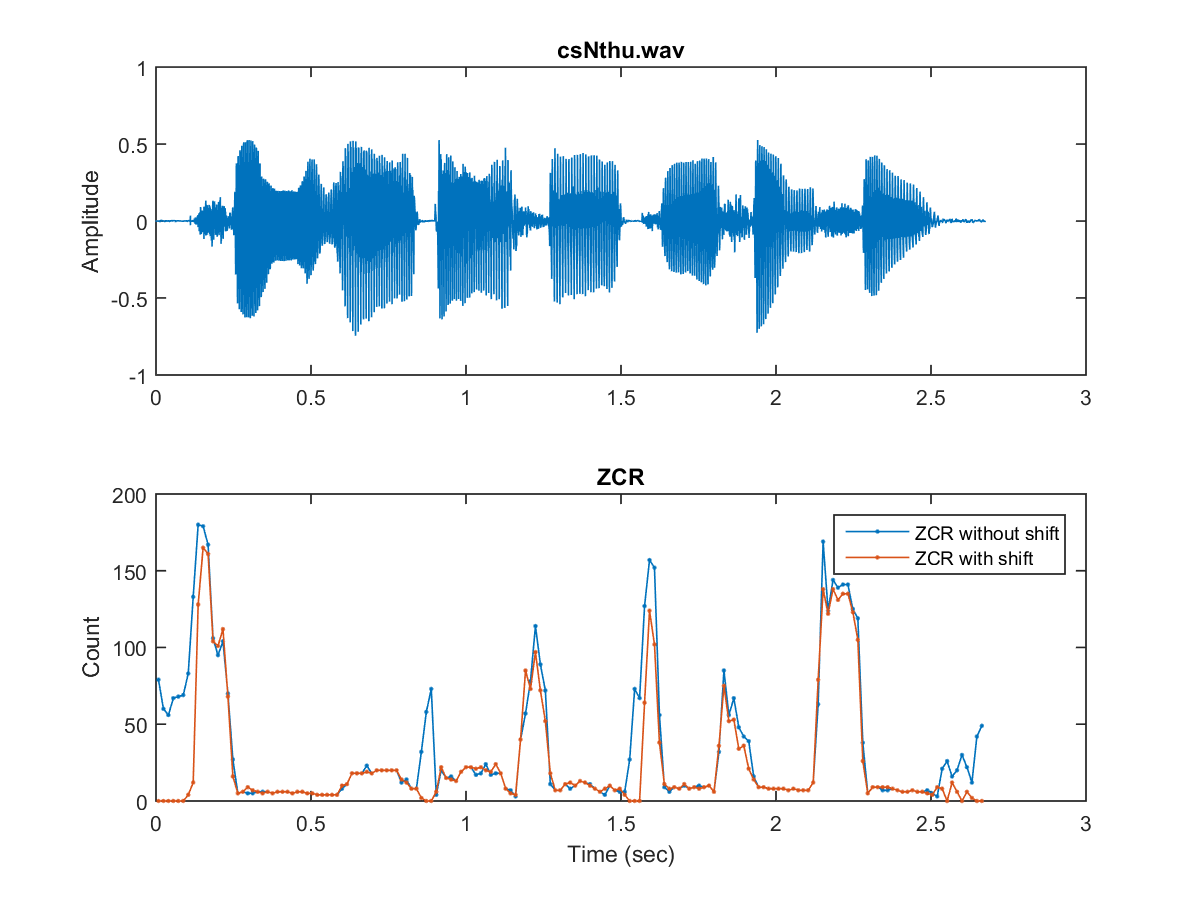In this example, the shift amount is equal to twice the maximal absolute sample value within the frame of the minimum volume. Therefore the ZCR of the silence is reduced drastically, making it easier to tell unvoiced sounds from silence ones using ZCR.

Moreover, we should be aware of the following facts:

1. If a sample is exactly located at zero, should we count it as zero crossing? Depending on the answer to this question, we have two methods for ZCR implementation.
2. Most ZCR computation is based on integer values of audio signals. If we want to do mean subtraction, the mean value should be rounded to the nearest integer too.
In the following, we use the above-mentioned two methods for ZCR computation of the wave file csNthu8b.wav:

Example 4: zcrOn8bit.mwaveFile='csNthu8b.wav'; frameSize=256; overlap=0; au=myAudioRead(waveFile); y=au.signal; fs=au.fs; nbits=au.nbits; y=y*2^nbits/2; frameMat=enframe(y, frameSize, overlap); frameNum=size(frameMat, 2); for i=1:frameNum frameMat(:,i)=frameMat(:,i)-round(mean(frameMat(:,i))); % Zero justification end zcr1=sum(frameMat(1:end-1, :).*frameMat(2:end, :)<0); % Method 1 zcr2=sum(frameMat(1:end-1, :).*frameMat(2:end, :)<=0); % Method 2 sampleTime=(1:length(y))/fs; frameNum=size(frameMat, 2); frameTime=((0:frameNum-1)*(frameSize-overlap)+0.5*frameSize)/fs; subplot(2,1,1); plot(sampleTime, y); ylabel(waveFile); subplot(2,1,2); plot(frameTime, zcr1, '.-', frameTime, zcr2, '.-'); title('ZCR'); xlabel('Time (sec)'); legend('Method 1', 'Method 2');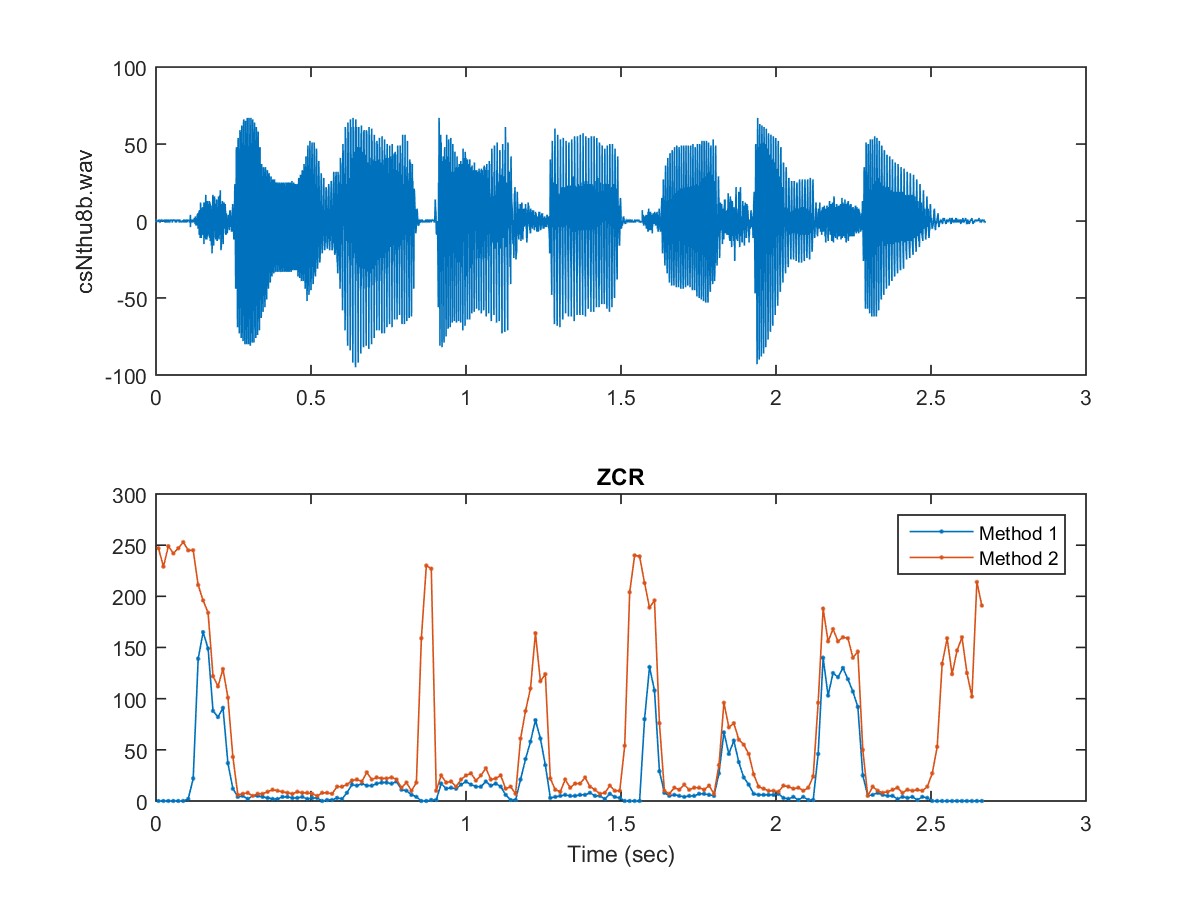From the above example, it is obvious that these two methods generate different ZCR curves. The first method does not count "zero sitting" as "zero crossing", there the corresponding ZCR values are smaller. Moreover, silence is likely to have low ZCR of method 1 and high ZCR for method 2 since there are likely to have many "zero sitting" in the silence region. However, this observation is only true for low sample rate (8 KHz in this case). For the same wave file with 16 KHz (csNthu.wav), the result is shown next:

Example 5: zcrOn16bit.mwaveFile='csNthu.wav'; frameSize=256; overlap=0; au=myAudioRead(waveFile); y=au.signal; fs=au.fs; nbits=au.nbits; y=y*2^nbits/2; frameMat=enframe(y, frameSize, overlap); frameNum=size(frameMat, 2); for i=1:frameNum frameMat(:,i)=frameMat(:,i)-round(mean(frameMat(:,i))); % Zero justification end zcr1=sum(frameMat(1:end-1, :).*frameMat(2:end, :)<0); % Method 1 zcr2=sum(frameMat(1:end-1, :).*frameMat(2:end, :)<=0); % Method 2 sampleTime=(1:length(y))/fs; frameTime=((0:frameNum-1)*(frameSize-overlap)+0.5*frameSize)/fs; subplot(2,1,1); plot(sampleTime, y); ylabel(waveFile); subplot(2,1,2); plot(frameTime, zcr1, '.-', frameTime, zcr2, '.-'); title('ZCR'); xlabel('Time (sec)'); legend('Method 1', 'Method 2');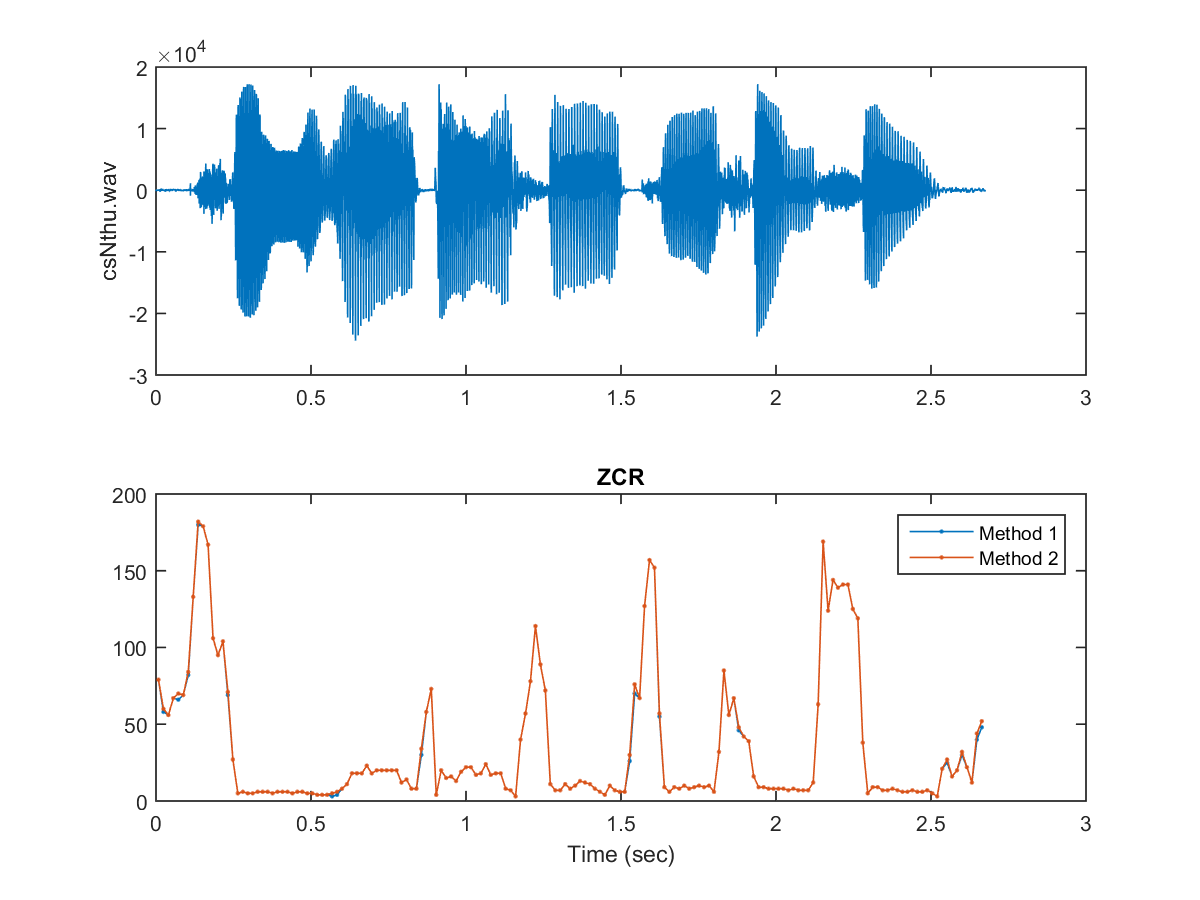If we want to detect the meaningful voice activity of a stream of audio signals, we need to perform end-point detection or speech detection. The most straightforward method for end-point detection is based on volume and ZCR. Please refer to the next chapter for more information.

Audio Signal Processing and Recognition (音訊處理與辨識)